# McGraw Hill Math Grade 3 Chapter 5 Lesson 3 Answer Key Dividing by 4

## McGraw-Hill Math Grade 3 Answer Key Chapter 5 Lesson 3 Dividing by 4

Solve

Divide. You can use objects to help.

Question 1.
12 ÷ 4 = 3

Question 2.
8 ÷ 4 = ________Explanation:
8 ÷ 4 = 2
Eight divided by four is two.

Question 3.
28 ÷ 4 = ___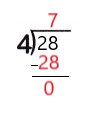Explanation:
28 ÷ 4 = 7
Twenty eight divided by four is seven.

Question 4.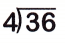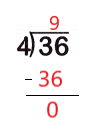Explanation:
36 ÷ 4 = 9
Thirty six  divided by four is nine.

Question 5.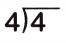Explanation:
4 ÷ 4 = 1
Four divided by four is one.

Question 6.Explanation:
0 ÷ 4 = 0
Zero divided by any number is 0.

Question 7.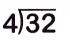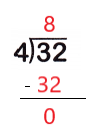Explanation:
32 ÷ 4 = 8
Thirty two divided by four is eight.

Question 8.
Tell which numbers are the dividend, divisor, and quotient in Exercise 4.
Dividend: ________ Divisor: ________ Quotient:
Dividend: 36
Divisor: 4
Quotient: 9

Explanation:
In exercise 4 the dividend is 36, divisor is 4 and the quotient is 9.

Question 9.
Evan has 16 eggs. He needs 4 eggs to make a batch of muffins. Does he have enough eggs to make 5 batches? Explain.
_____ ÷ ___ = ________ batches